# spectrogram

Spectrogram using short-time Fourier transform

## Syntax

``s = spectrogram(x)``
``s = spectrogram(x,window)``
``s = spectrogram(x,window,noverlap)``
``s = spectrogram(x,window,noverlap,nfft)``
``````[s,w,t] = spectrogram(___)``````
``````[s,f,t] = spectrogram(___,fs)``````
``````[s,w,t] = spectrogram(x,window,noverlap,w)``````
``````[s,f,t] = spectrogram(x,window,noverlap,f,fs)``````
``[___,ps] = spectrogram(___)``
``[___] = spectrogram(___,'reassigned')``
``````[___,ps,fc,tc] = spectrogram(___)``````
``[___] = spectrogram(___,freqrange)``
``[___] = spectrogram(___,Name,Value)``
``[___] = spectrogram(___,spectrumtype)``
``spectrogram(___)``
``spectrogram(___,freqloc)``

## Description

example

````s = spectrogram(x)` returns the short-time Fourier transform of the input signal, `x`. Each column of `s` contains an estimate of the short-term, time-localized frequency content of `x`.```
````s = spectrogram(x,window)` uses `window` to divide the signal into segments and perform windowing.```

example

````s = spectrogram(x,window,noverlap)` uses `noverlap` samples of overlap between adjoining segments.```

example

````s = spectrogram(x,window,noverlap,nfft)` uses `nfft` sampling points to calculate the discrete Fourier transform.```
``````[s,w,t] = spectrogram(___)``` returns a vector of normalized frequencies, `w`, and a vector of time instants, `t`, at which the spectrogram is computed. This syntax can include any combination of input arguments from previous syntaxes.```

example

``````[s,f,t] = spectrogram(___,fs)``` returns a vector of cyclical frequencies, `f`, expressed in terms of the sample rate, `fs`. `fs` must be the fifth input to `spectrogram`. To input a sample rate and still use the default values of the preceding optional arguments, specify these arguments as empty, `[]`.```

example

``````[s,w,t] = spectrogram(x,window,noverlap,w)``` returns the spectrogram at the normalized frequencies specified in `w`.```
``````[s,f,t] = spectrogram(x,window,noverlap,f,fs)``` returns the spectrogram at the cyclical frequencies specified in `f`.```
````[___,ps] = spectrogram(___)` also returns a matrix, `ps`, containing an estimate of the power spectral density (PSD) or the power spectrum of each segment.```

example

````[___] = spectrogram(___,'reassigned')` reassigns each PSD or power spectrum estimate to the location of its center of energy. If your signal contains well-localized temporal or spectral components, then this option generates a sharper spectrogram.```

example

``````[___,ps,fc,tc] = spectrogram(___)``` also returns two matrices, `fc` and `tc`, containing the frequency and time of the center of energy of each PSD or power spectrum estimate.```
````[___] = spectrogram(___,freqrange)` returns the PSD or power spectrum estimate over the frequency range specified by `freqrange`. Valid options for `freqrange` are `'onesided'`, `'twosided'`, and `'centered'`.```

example

````[___] = spectrogram(___,Name,Value)` specifies additional options using name-value pair arguments. Options include the minimum threshold and output time dimension.```
````[___] = spectrogram(___,spectrumtype)` returns PSD estimates if `spectrumtype` is specified as `'psd'` and returns power spectrum estimates if `spectrumtype` is specified as `'power'`.```

example

````spectrogram(___)` with no output arguments plots the spectrogram in the current figure window.```

example

````spectrogram(___,freqloc)` specifies the axis on which to plot the frequency.```

## Examples

collapse all

Generate ${N}_{x}=1024$ samples of a signal that consists of a sum of sinusoids. The normalized frequencies of the sinusoids are $2\pi /5$ rad/sample and $4\pi /5$ rad/sample. The higher frequency sinusoid has 10 times the amplitude of the other sinusoid.

```N = 1024; n = 0:N-1; w0 = 2*pi/5; x = sin(w0*n)+10*sin(2*w0*n);```

Compute the short-time Fourier transform using the function defaults. Plot the spectrogram.

```s = spectrogram(x); spectrogram(x,'yaxis')```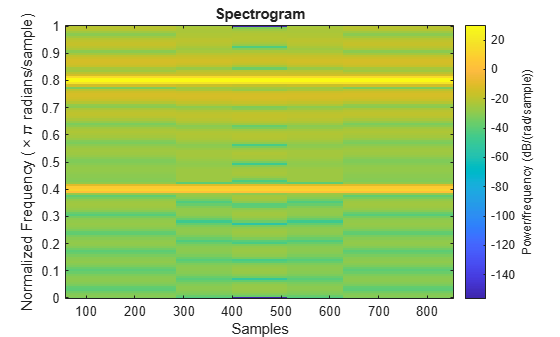Repeat the computation.

• Divide the signal into sections of length $nsc=⌊{N}_{x}/4.5⌋$.

• Window the sections using a Hamming window.

• Specify 50% overlap between contiguous sections.

• To compute the FFT, use $\mathrm{max}\left(256,{2}^{p}\right)$ points, where $p=⌈{\mathrm{log}}_{2}nsc⌉$.

Verify that the two approaches give identical results.

```Nx = length(x); nsc = floor(Nx/4.5); nov = floor(nsc/2); nff = max(256,2^nextpow2(nsc)); t = spectrogram(x,hamming(nsc),nov,nff); maxerr = max(abs(abs(t(:))-abs(s(:))))```
```maxerr = 0 ```

Divide the signal into 8 sections of equal length, with 50% overlap between sections. Specify the same FFT length as in the preceding step. Compute the short-time Fourier transform and verify that it gives the same result as the previous two procedures.

```ns = 8; ov = 0.5; lsc = floor(Nx/(ns-(ns-1)*ov)); t = spectrogram(x,lsc,floor(ov*lsc),nff); maxerr = max(abs(abs(t(:))-abs(s(:))))```
```maxerr = 0 ```

Generate a quadratic chirp, `x`, sampled at 1 kHz for 2 seconds. The frequency of the chirp is 100 Hz initially and crosses 200 Hz at `t` = 1 s.

```t = 0:0.001:2; x = chirp(t,100,1,200,'quadratic');```

Compute and display the spectrogram of `x`.

• Divide the signal into sections of length 128, windowed with a Hamming window.

• Specify 120 samples of overlap between adjoining sections.

• Evaluate the spectrum at $⌊128/2+1⌋=65$ frequencies and $⌊\left(length\left(x\right)-120\right)/\left(128-120\right)⌋=235$ time bins.

`spectrogram(x,128,120,128,1e3)`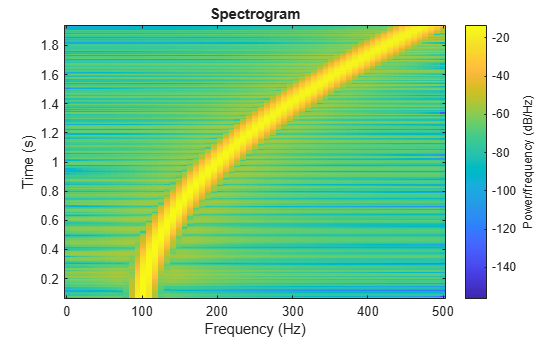Replace the Hamming window with a Blackman window. Decrease the overlap to 60 samples. Plot the time axis so that its values increase from top to bottom.

```spectrogram(x,blackman(128),60,128,1e3) ax = gca; ax.YDir = 'reverse';```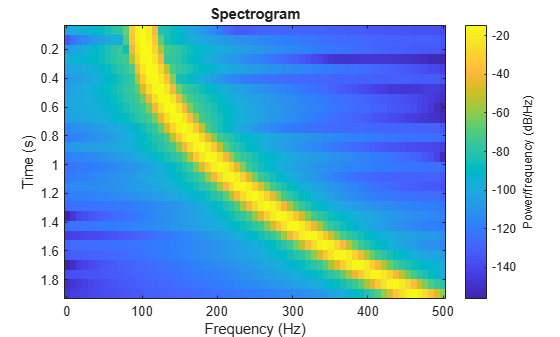Compute and display the PSD of each segment of a quadratic chirp that starts at 100 Hz and crosses 200 Hz at t = 1 second. Specify a sample rate of 1 kHz, a segment length of 128 samples, and an overlap of 120 samples. Use 128 DFT points and the default Hamming window.

```fs = 1000; t = 0:1/fs:2; x = chirp(t,100,1,200,'quadratic'); spectrogram(x,128,120,128,fs,'yaxis') title('Quadratic Chirp')```Compute and display the PSD of each segment of a linear chirp sampled at 1 kHz that starts at DC and crosses 150 Hz at t = 1 second. Specify a segment length of 256 samples and an overlap of 250 samples. Use the default Hamming window and 256 DFT points.

```x = chirp(t,0,1,150); spectrogram(x,256,250,256,fs,'yaxis') title('Linear Chirp')```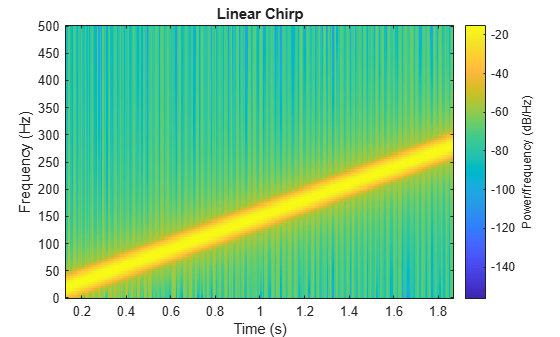Compute and display the PSD of each segment of a logarithmic chirp sampled at 1 kHz that starts at 20 Hz and crosses 60 Hz at t = 1 second. Specify a segment length of 256 samples and an overlap of 250 samples. Use the default Hamming window and 256 DFT points.

```x = chirp(t,20,1,60,'logarithmic'); spectrogram(x,256,250,[],fs,'yaxis') title('Logarithmic Chirp')```Use a logarithmic scale for the frequency axis. The spectrogram becomes a line.

```ax = gca; ax.YScale = 'log';```Use the `spectrogram` function to measure and track the instantaneous frequency of a signal.

Generate a quadratic chirp sampled at 1 kHz for two seconds. Specify the chirp so that its frequency is initially 100 Hz and increases to 200 Hz after one second.

```fs = 1000; t = 0:1/fs:2-1/fs; y = chirp(t,100,1,200,'quadratic');```

Estimate the spectrum of the chirp using the short-time Fourier transform implemented in the `spectrogram` function. Divide the signal into sections of length 100, windowed with a Hamming window. Specify 80 samples of overlap between adjoining sections and evaluate the spectrum at $⌊100/2+1⌋=51$ frequencies.

`spectrogram(y,100,80,100,fs,'yaxis')`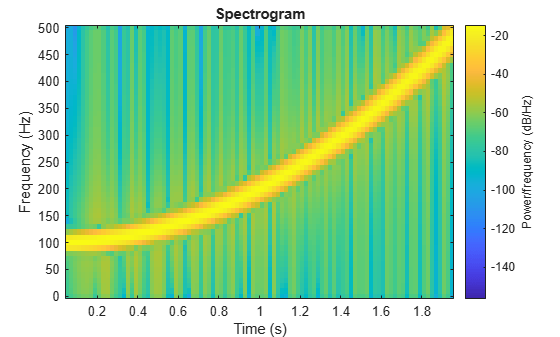Track the chirp frequency by finding the time-frequency ridge with highest energy across the $⌊\left(2000-80\right)/\left(100-80\right)⌋=96$ time points. Overlay the instantaneous frequency on the spectrogram plot.

```[~,f,t,p] = spectrogram(y,100,80,100,fs); [fridge,~,lr] = tfridge(p,f); hold on plot3(t,fridge,abs(p(lr)),'LineWidth',4) hold off```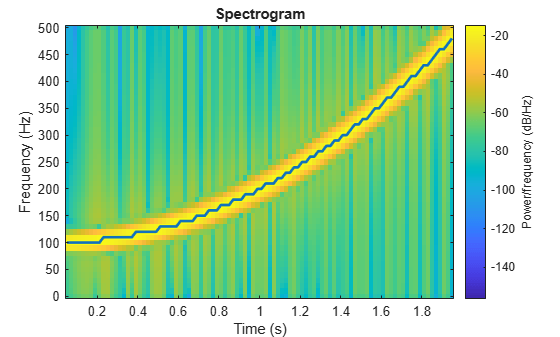Generate 512 samples of a chirp with sinusoidally varying frequency content.

```N = 512; n = 0:N-1; x = exp(1j*pi*sin(8*n/N)*32);```

Compute the centered two-sided short-time Fourier transform of the chirp. Divide the signal into 32-sample segments with 16-sample overlap. Specify 64 DFT points. Plot the spectrogram.

```[scalar,fs,ts] = spectrogram(x,32,16,64,'centered'); spectrogram(x,32,16,64,'centered','yaxis')```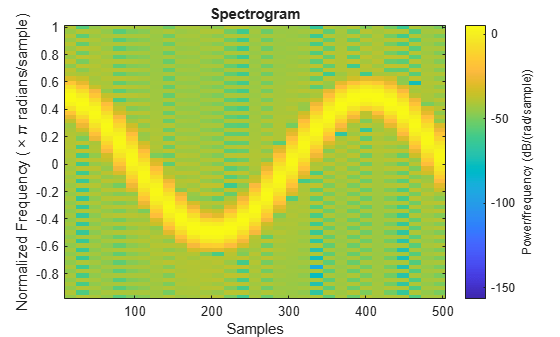Obtain the same result by computing the spectrogram on 64 equispaced frequencies over the interval $\left(-\pi ,\pi \right]$. The `'centered'` option is not necessary.

```fintv = -pi+pi/32:pi/32:pi; [vector,fv,tv] = spectrogram(x,32,16,fintv); spectrogram(x,32,16,fintv,'yaxis')```Generate a chirp signal sampled for 2 seconds at 1 kHz. Specify the chirp so that its frequency is initially 100 Hz and increases to 200 Hz after 1 second.

```Fs = 1000; t = 0:1/Fs:2; y = chirp(t,100,1,200,'quadratic');```

Estimate the reassigned spectrogram of the signal.

• Divide the signal into sections of length 128, windowed with a Kaiser window with shape parameter $\beta =18$.

• Specify 120 samples of overlap between adjoining sections.

• Evaluate the spectrum at $⌊128/2⌋=65$ frequencies and $⌊\left(length\left(x\right)-120\right)/\left(128-120\right)⌋=235$ time bins.

`spectrogram(y,kaiser(128,18),120,128,Fs,'reassigned','yaxis')`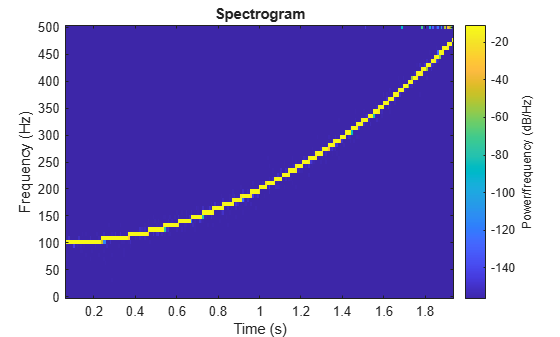Generate a chirp signal sampled for 2 seconds at 1 kHz. Specify the chirp so that its frequency is initially 100 Hz and increases to 200 Hz after 1 second.

```Fs = 1000; t = 0:1/Fs:2; y = chirp(t,100,1,200,'quadratic');```

Estimate the time-dependent power spectral density (PSD) of the signal.

• Divide the signal into sections of length 128, windowed with a Kaiser window with shape parameter $\beta =18$.

• Specify 120 samples of overlap between adjoining sections.

• Evaluate the spectrum at $⌊128/2⌋=65$ frequencies and $⌊\left(length\left(x\right)-120\right)/\left(128-120\right)⌋=235$ time bins.

Output the frequency and time of the center of gravity of each PSD estimate. Set to zero those elements of the PSD smaller than $-30$ dB.

```[~,~,~,pxx,fc,tc] = spectrogram(y,kaiser(128,18),120,128,Fs, ... 'MinThreshold',-30);```

Plot the nonzero elements as functions of the center-of-gravity frequencies and times.

`plot(tc(pxx>0),fc(pxx>0),'.')`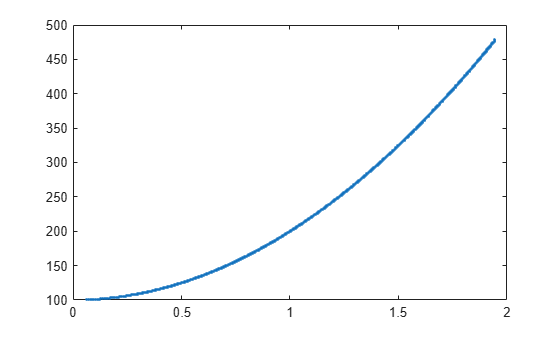Generate a signal sampled at 1024 Hz for 2 seconds.

```nSamp = 2048; Fs = 1024; t = (0:nSamp-1)'/Fs;```

During the first second, the signal consists of a 400 Hz sinusoid and a concave quadratic chirp. Specify the chirp so that it is symmetric about the interval midpoint, starting and ending at a frequency of 250 Hz and attaining a minimum of 150 Hz.

```t1 = t(1:nSamp/2); x11 = sin(2*pi*400*t1); x12 = chirp(t1-t1(nSamp/4),150,nSamp/Fs,1750,'quadratic'); x1 = x11+x12;```

The rest of the signal consists of two linear chirps of decreasing frequency. One chirp has an initial frequency of 250 Hz that decreases to 100 Hz. The other chirp has an initial frequency of 400 Hz that decreases to 250 Hz.

```t2 = t(nSamp/2+1:nSamp); x21 = chirp(t2,400,nSamp/Fs,100); x22 = chirp(t2,550,nSamp/Fs,250); x2 = x21+x22;```

Add white Gaussian noise to the signal. Specify a signal-to-noise ratio of 20 dB. Reset the random number generator for reproducible results.

```SNR = 20; rng('default') sig = [x1;x2]; sig = sig + randn(size(sig))*std(sig)/db2mag(SNR);```

Compute and plot the spectrogram of the signal. Specify a Kaiser window of length 63 with a shape parameter $\beta =17$, 10 fewer samples of overlap between adjoining sections, and an FFT length of 256.

```nwin = 63; wind = kaiser(nwin,17); nlap = nwin-10; nfft = 256; spectrogram(sig,wind,nlap,nfft,Fs,'yaxis')```Threshold the spectrogram so that any elements with values smaller than the SNR are set to zero.

`spectrogram(sig,wind,nlap,nfft,Fs,'MinThreshold',-SNR,'yaxis')`Reassign each PSD estimate to the location of its center of energy.

`spectrogram(sig,wind,nlap,nfft,Fs,'reassign','yaxis')`Threshold the reassigned spectrogram so that any elements with values smaller than the SNR are set to zero.

`spectrogram(sig,wind,nlap,nfft,Fs,'reassign','MinThreshold',-SNR,'yaxis')`Load an audio signal that contains two decreasing chirps and a wideband splatter sound. Compute the short-time Fourier transform. Divide the waveform into 400-sample segments with 300-sample overlap. Plot the spectrogram.

```load splat % To hear, type soundsc(y,Fs) sg = 400; ov = 300; spectrogram(y,sg,ov,[],Fs,'yaxis') colormap bone```Use the `spectrogram` function to output the power spectral density (PSD) of the signal.

`[s,f,t,p] = spectrogram(y,sg,ov,[],Fs);`

Track the two chirps using the `medfreq` function. To find the stronger, low-frequency chirp, restrict the search to frequencies above 100 Hz and to times before the start of the wideband sound.

```f1 = f > 100; t1 = t < 0.75; m1 = medfreq(p(f1,t1),f(f1));```

To find the faint high-frequency chirp, restrict the search to frequencies above 2500 Hz and to times between 0.3 seconds and 0.65 seconds.

```f2 = f > 2500; t2 = t > 0.3 & t < 0.65; m2 = medfreq(p(f2,t2),f(f2));```

Overlay the result on the spectrogram. Divide the frequency values by 1000 to express them in kHz.

```hold on plot(t(t1),m1/1000,'linewidth',4) plot(t(t2),m2/1000,'linewidth',4) hold off```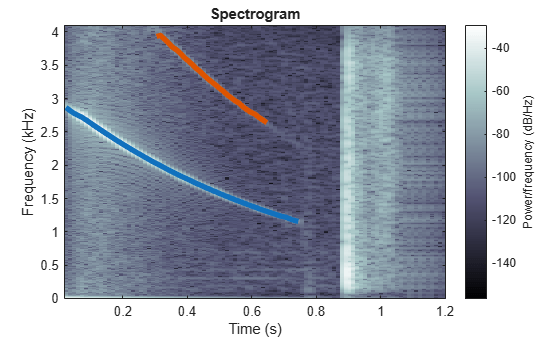Generate two seconds of a signal sampled at 10 kHz. Specify the instantaneous frequency of the signal as a triangular function of time.

```fs = 10e3; t = 0:1/fs:2; x1 = vco(sawtooth(2*pi*t,0.5),[0.1 0.4]*fs,fs);```

Compute and plot the spectrogram of the signal. Use a Kaiser window of length 256 and shape parameter $\beta =5$. Specify 220 samples of section-to-section overlap and 512 DFT points. Plot the frequency on the y-axis. Use the default colormap and view.

`spectrogram(x1,kaiser(256,5),220,512,fs,'yaxis')`Change the view to display the spectrogram as a waterfall plot. Set the colormap to `bone`.

```colormap bone view(-45,65)```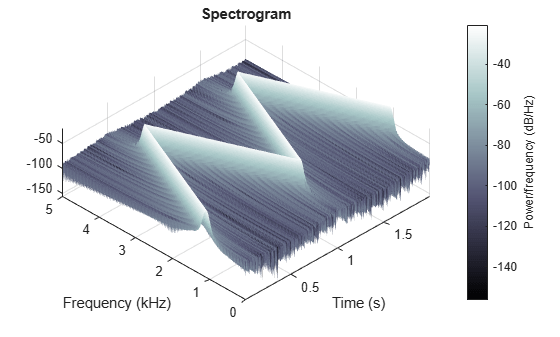## Input Arguments

collapse all

Input signal, specified as a row or column vector.

Example: `cos(pi/4*(0:159))+randn(1,160)` specifies a sinusoid embedded in white Gaussian noise.

Data Types: `single` | `double`
Complex Number Support: Yes

Window, specified as an integer or as a row or column vector. Use `window` to divide the signal into segments:

• If `window` is an integer, then `spectrogram` divides `x` into segments of length `window` and windows each segment with a Hamming window of that length.

• If `window` is a vector, then `spectrogram` divides `x` into segments of the same length as the vector and windows each segment using `window`.

If the length of `x` cannot be divided exactly into an integer number of segments with `noverlap` overlapping samples, then `x` is truncated accordingly.

If you specify `window` as empty, then `spectrogram` uses a Hamming window such that `x` is divided into eight segments with `noverlap` overlapping samples.

For a list of available windows, see Windows.

Example: `hann(N+1)` and `(1-cos(2*pi*(0:N)'/N))/2` both specify a Hann window of length `N` + 1.

Number of overlapped samples, specified as a positive integer.

• If `window` is scalar, then `noverlap` must be smaller than `window`.

• If `window` is a vector, then `noverlap` must be smaller than the length of `window`.

If you specify `noverlap` as empty, then `spectrogram` uses a number that produces 50% overlap between segments. If the segment length is unspecified, the function sets `noverlap` to Nx/4.5⌋, where Nx is the length of the input signal and the ⌊⌋ symbols denote the floor function.

Number of DFT points, specified as a positive integer scalar. If you specify `nfft` as empty, then `spectrogram` sets the parameter to max(256,2p), where p = ⌈log2 Nw, the ⌈⌉ symbols denote the ceiling function, and

• Nw = `window` if `window` is a scalar.

• Nw = `length(window)` if `window` is a vector.

Normalized frequencies, specified as a vector. `w` must have at least two elements, because otherwise the function interprets it as `nfft`. Normalized frequencies are in rad/sample.

Example: `pi./[2 4]`

Cyclical frequencies, specified as a vector. `f` must have at least two elements, because otherwise the function interprets it as `nfft`. The units of `f` are specified by the sample rate, `fs`.

Sample rate, specified as a positive scalar. The sample rate is the number of samples per unit time. If the unit of time is seconds, then the sample rate is in Hz.

Frequency range for the PSD estimate, specified as `'onesided'`, `'twosided'`, or `'centered'`. For real-valued signals, the default is `'onesided'`. For complex-valued signals, the default is `'twosided'`, and specifying `'onesided'` results in an error.

• `'onesided'` — returns the one-sided spectrogram of a real input signal. If `nfft` is even, then `ps` has `nfft`/2 + 1 rows and is computed over the interval [0, π] rad/sample. If `nfft` is odd, then `ps` has (`nfft` + 1)/2 rows and the interval is [0, π) rad/sample. If you specify `fs`, then the intervals are respectively [0, `fs`/2] cycles/unit time and [0, `fs`/2) cycles/unit time.

• `'twosided'` — returns the two-sided spectrogram of a real or complex signal. `ps` has `nfft` rows and is computed over the interval [0, 2π) rad/sample. If you specify `fs`, then the interval is [0, `fs`) cycles/unit time.

• `'centered'` — returns the centered two-sided spectrogram of a real or complex signal. `ps` has `nfft` rows. If `nfft` is even, then `ps` is computed over the interval (–ππ] rad/sample. If `nfft` is odd, then `ps` is computed over (–ππ) rad/sample. If you specify `fs`, then the intervals are respectively (–`fs`/2, `fs`/2] cycles/unit time and (–`fs`/2, `fs`/2) cycles/unit time.

Power spectrum scaling, specified as `'psd'` or `'power'`.

• Omitting `spectrumtype`, or specifying `'psd'`, returns the power spectral density.

• Specifying `'power'` scales each estimate of the PSD by the equivalent noise bandwidth of the window. The result is an estimate of the power at each frequency. If the `'reassigned'` option is on, the function integrates the PSD over the width of each frequency bin before reassigning.

Frequency display axis, specified as `'xaxis'` or `'yaxis'`.

• `'xaxis'` — displays frequency on the x-axis and time on the y-axis.

• `'yaxis'` — displays frequency on the y-axis and time on the x-axis.

This argument is ignored if you call `spectrogram` with output arguments.

### Name-Value Pair Arguments

Specify optional comma-separated pairs of `Name,Value` arguments. `Name` is the argument name and `Value` is the corresponding value. `Name` must appear inside quotes. You can specify several name and value pair arguments in any order as `Name1,Value1,...,NameN,ValueN`.

Example: `spectrogram(x,100,'OutputTimeDimension','downrows')` divides `x` into segments of length 100 and windows each segment with a Hamming window of that length The output of the spectrogram has time dimension down the rows.

Threshold, specified as the comma-separated pair consisting of `MinThreshold` and a real scalar expressed in decibels. `spectrogram` sets to zero those elements of `s` such that 10 log10(`s`) ≤ `thresh`.

Output time dimension, specified as the comma-separated pair consisting of `OutputTimeDimension` and `acrosscolumns` or `downrows`. Set this value to `downrows`, if you want the time dimension of `s`, `ps`, `fc`, and `tc` down the rows and the frequency dimension along the columns. Set this value to `acrosscolumns`, if you want the time dimension of `s`, `ps`, `fc`, and `tc` across the columns and frequency dimension along the rows. This input is ignored if the function is called without output arguments.

## Output Arguments

collapse all

Short-time Fourier transform, returned as a matrix. Time increases across the columns of `s` and frequency increases down the rows, starting from zero.

• If `x` is a signal of length Nx, then `s` has k columns, where

• k = ⌊(Nx – `noverlap`)/(`window` – `noverlap`)⌋ if `window` is a scalar.

• k = ⌊(Nx – `noverlap`)/(`length(window)` – `noverlap`)⌋ if `window` is a vector.

• If `x` is real and `nfft` is even, then `s` has (`nfft`/2 + 1) rows.

• If `x` is real and `nfft` is odd, then `s` has (`nfft` + 1)/2 rows.

• If `x` is complex, then `s` has `nfft` rows.

`s` is not affected by the `'reassigned'` option.

Normalized frequencies, returned as a vector. `w` has a length equal to the number of rows of `s`.

Time instants, returned as a vector. The time values in `t` correspond to the midpoint of each segment.

Cyclical frequencies, returned as a vector. `f` has a length equal to the number of rows of `s`.

Power spectral density (PSD) or power spectrum, returned as a matrix.

• If `x` is real, then `ps` contains the one-sided modified periodogram estimate of the PSD or power spectrum of each segment.

• If `x` is complex, or if you specify a vector of frequencies, then `ps` contains the two-sided modified periodogram estimate of the PSD or power spectrum of each segment.

Center-of-energy frequencies and times, returned as matrices of the same size as the short-time Fourier transform. If you do not specify a sample rate, then the elements of `fc` are returned as normalized frequencies.

## Tips

If a short-time Fourier transform has zeros, its conversion to decibels results in negative infinities that cannot be plotted. To avoid this potential difficulty, `spectrogram` adds `eps` to the short-time Fourier transform when you call it with no output arguments.

 Oppenheim, Alan V., Ronald W. Schafer, and John R. Buck. Discrete-Time Signal Processing. 2nd Ed. Upper Saddle River, NJ: Prentice Hall, 1999.

 Rabiner, Lawrence R., and Ronald W. Schafer. Digital Processing of Speech Signals. Englewood Cliffs, NJ: Prentice-Hall, 1978.

 Chassande-Motin, Éric, François Auger, and Patrick Flandrin. “Reassignment.” In Time-Frequency Analysis: Concepts and Methods. Edited by Franz Hlawatsch and François Auger. London: ISTE/John Wiley and Sons, 2008.

 Fulop, Sean A., and Kelly Fitz. “Algorithms for computing the time-corrected instantaneous frequency (reassigned) spectrogram, with applications.” Journal of the Acoustical Society of America. Vol. 119, January 2006, pp. 360–371.